Function Repository Resource:

# CellBoundingRectangle

Get the bounding rectangle coordinates for a cell as it appears in a notebook

Contributed by: Alexey Popkov
 ResourceFunction["CellBoundingRectangle"][cellObj] gives the bounding rectangle coordinates for the contents of the cell cellObj. ResourceFunction["CellBoundingRectangle"][cellObj,Full] gives the coordinates of a rectangle that fills out the whole cell cellObj. ResourceFunction["CellBoundingRectangle"][cellObj,spec,ImageSize] returns the bounding box information suitable for immediate use as a value for ImageSize.

## Details

ResourceFunction["CellBoundingRectangle"] is intended for use within the Notebook interface.
The basic usage syntax is ResourceFunction["CellBoundingRectangle"][cellObj,spec], where spec can be either CellContents or Full.
ResourceFunction["CellBoundingRectangle"][cellObj] is a shorthand for ResourceFunction["CellBoundingRectangle"][cellObj,CellContents].
ResourceFunction["CellBoundingRectangle"][cellObj,spec] returns coordinates in printer's points in the form {{xmin,ymin},{xmax,ymax}}, where y coordinates run from top to bottom relative to the Notebook window.
ResourceFunction["CellBoundingRectangle"][cellObj,spec,ImageSize] returns bounding box size in printer's points in the form {width,height}, taking into account current Magnification setting for cellObj. This value is suitable for immediate use as a value for ImageSize option of functions such as Pane, Graphics, etc.

## Examples

### Basic Examples (6)

Get the bounding rectangle coordinates of the contents of the current cell:

 In:=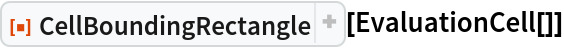Out=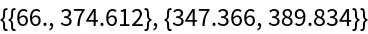Get the bounding rectangle coordinates of a rectangle that fills out the whole internal area of the current cell:

 In:=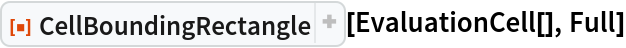Out=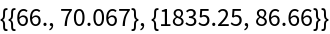Generate an output cell with some text:

 In:=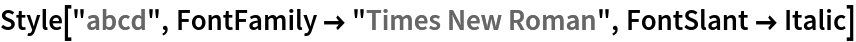Out=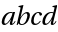Get the bounding box size of the text in printer's points:

 In:=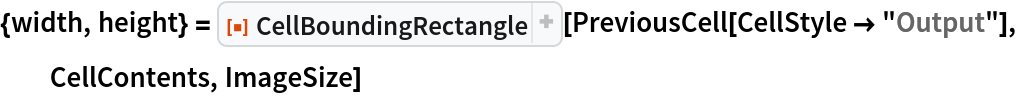Out=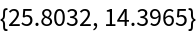Take some image object and adjust its size manually:

 In:=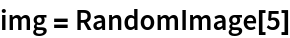Out=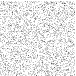Get the current bounding box size of the object and specify it as ImageSize:

 In:=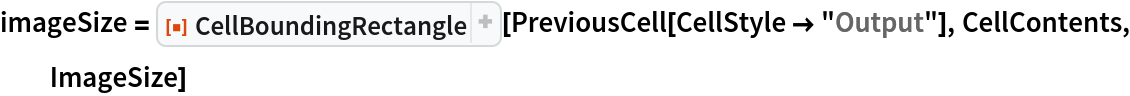Out=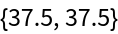In:=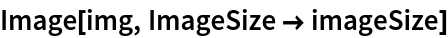Out=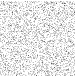First, produce an output cell:

 In:=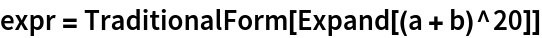Out=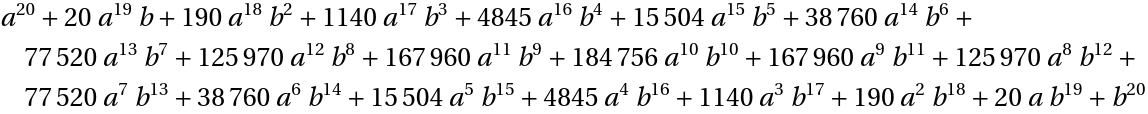Get the current absolute wrapping width of the produced cell and specify it as ImageFormattingWidth for Rasterize in order to reproduce the current view:

 In:=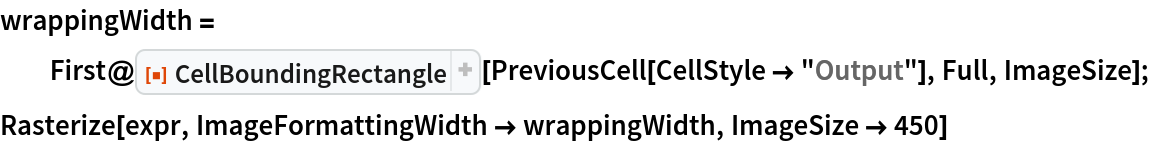Out=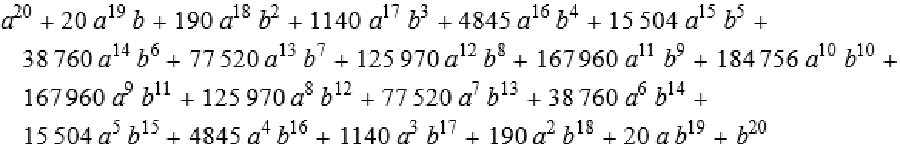Specify the current absolute wrapping width of the output cell as ImageSize for Pane, in order to reproduce the current wrapping independently of the WindowSize:

 In:=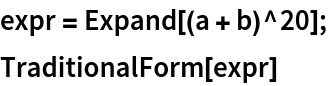Out=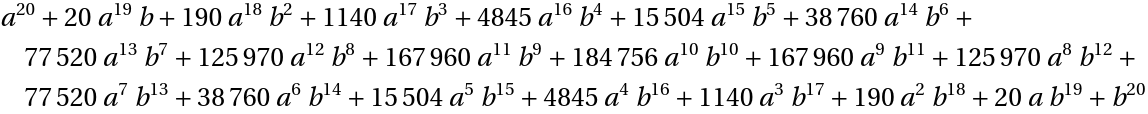In:=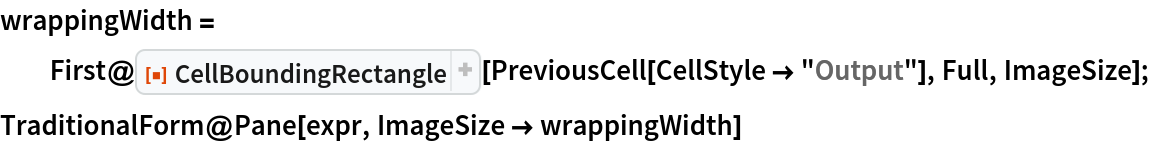Out=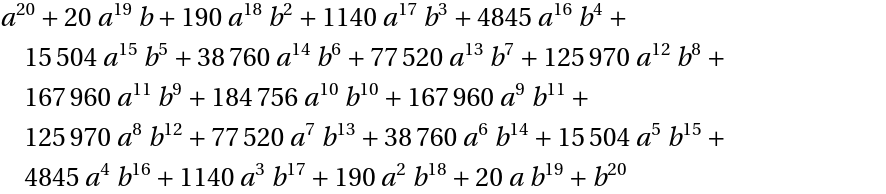### Applications (2)

Export a Wolfram Language expression exactly as it appears on the screen. First, generate some expression as an output cell:

 In:=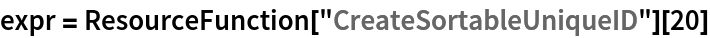Out=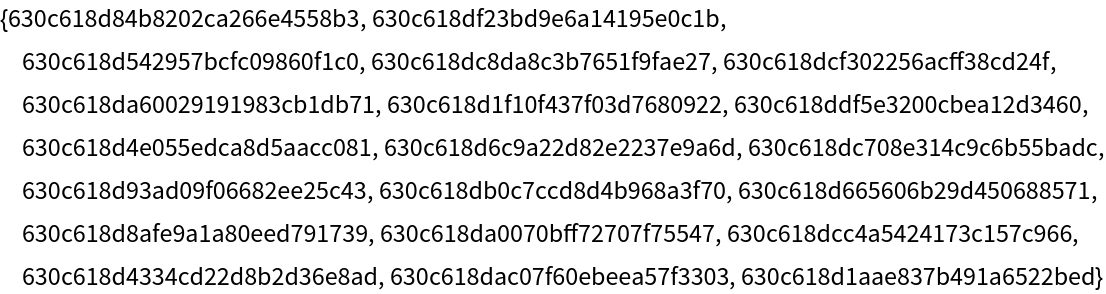Adjust the window width of the current notebook in order to obtain the wrapping you wish. Then obtain the current wrappingWidth of the output cell, and export the expression as Pane with ImageSizewrappingWidth:

 In:=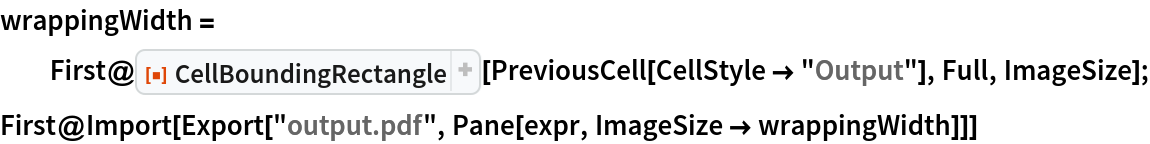Out=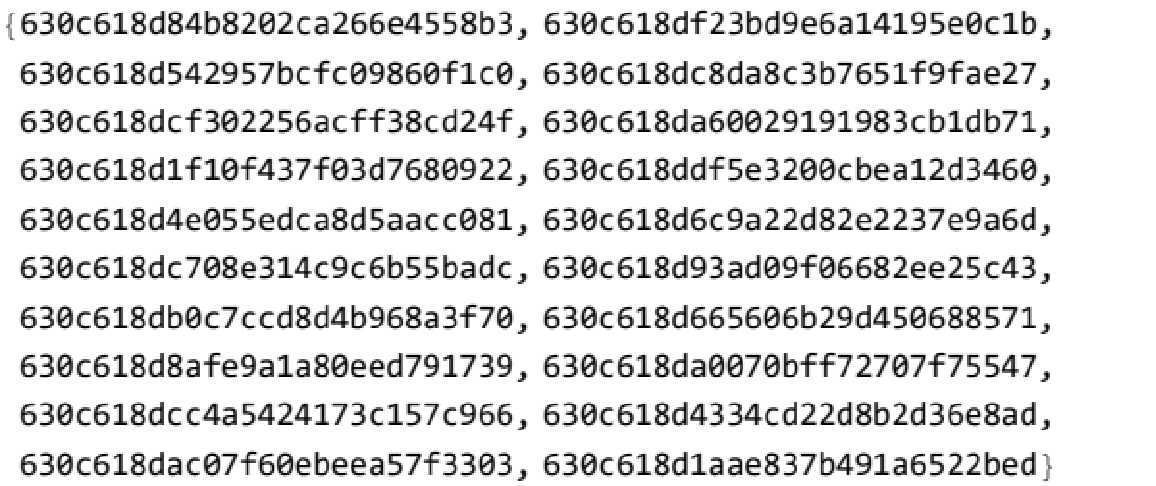### Properties and Relations (2)

Define a region using Annotation:

 In:=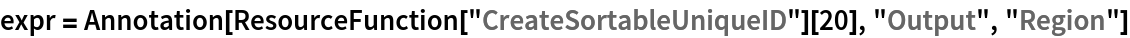Out=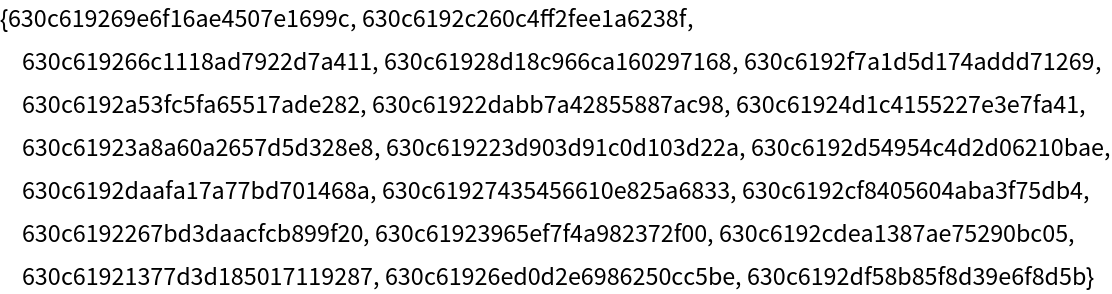CellBoundingRectangle returns the bounding rectangle for the cell as it currently appears on the screen:

 In:=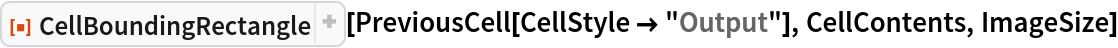Out=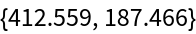Rasterize[expr,"Regions"] also returns the bounding rectangle for the region defined by Annotation, taking fixed values for some Notebook and Cell options, including PageWidth and Magnification:

 In:=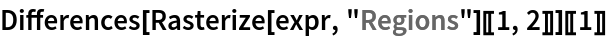Out=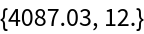By setting these options explicitly, we can partially reproduce the result of Rasterize with CellBoundingRectangle:

 In:=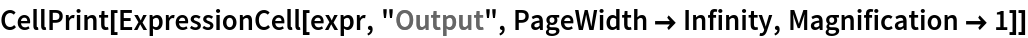Out=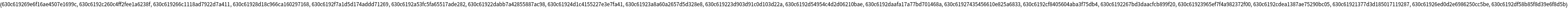In:=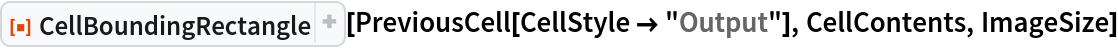Out=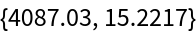AbsoluteCurrentValue[cellObj,CellSize] also returns the bounding rectangle information for the contents of the cell cellObj, but the width differs from that returned by CellBoundingRectangle[cellObj,CellContents,ImageSize] by a fixed amount with the given value of the Magnification option:

 In:=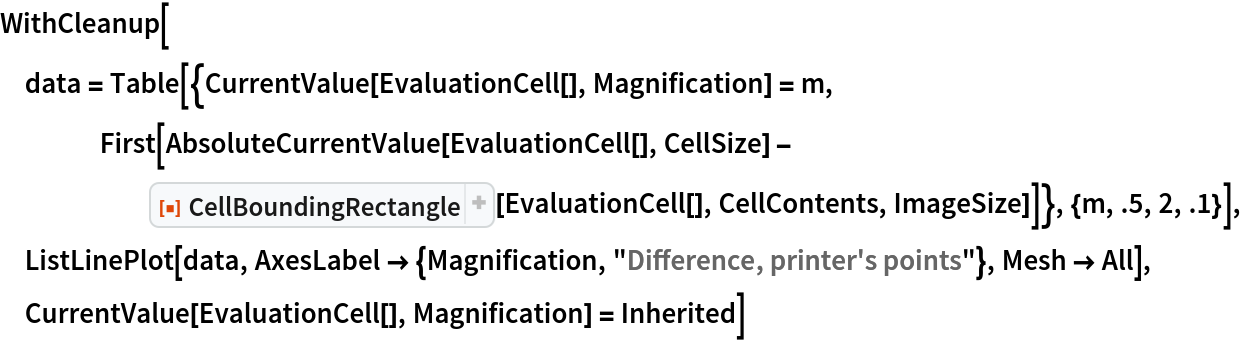Out=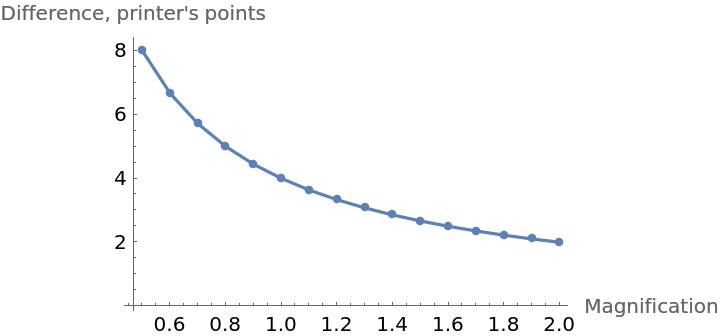### Possible Issues (1)

Cell heights returned by CellBoundingRectangle with different second arguments may differ:

 In:=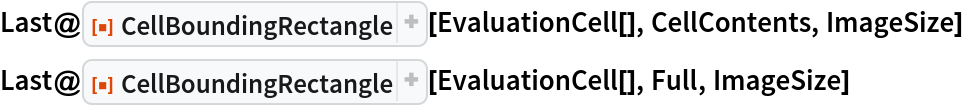Out=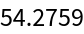Out=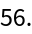AlexeyPopkov

## Version History

• 2.0.0 – 09 September 2022
• 1.0.0 – 23 August 2022

## Author Notes

CellBoundingRectangle isn't intended to be used within Dynamic.

Version 2.0.0 features a breaking change to the way the rectangle is calculated. Now, the form CellBoundingRectangle[cellObj,Full] returns the coordinates of a rectangle, which fills out the whole cellObj. In the previous version of the function it returned the coordinates of a square, that fills out the whole cellObj. Correspondingly, the form CellBoundingRectangle[cellObj,Full,ImageSize] now returns the image size of that rectangle.